# Critical point

(diff) ← Older revision | Latest revision (diff) | Newer revision → (diff)

For an analytic function, a critical point of orderis a pointof the complex plane at whichis regular but its derivative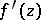has a zero of order, whereis a natural number. In other words, a critical point is defined by the conditions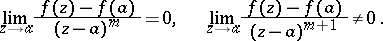A critical point at infinity,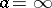, of order, for a functionwhich is regular at infinity, is defined by the conditionsUnder the analytic mapping, the angle between two curves emanating from a critical point of orderis increased by a factor. Ifis regarded as the complex potential of some planar flow of an incompressible liquid, a critical point is characterized by the property that through it pass not one but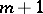stream lines, and the velocity of the flow at a critical point vanishes. In terms of the inverse function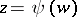(i.e. the function for which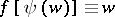), a critical point is an algebraic branch point of order.

A pointof a complex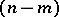-dimensional irreducible analytic setthe latter being defined in a neighbourhoodofin the complex spaceby the conditions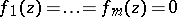, whereare holomorphic functions onincomplex variables,, is called a critical point if the rank of the Jacobian matrix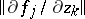,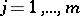,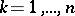, is less than. The other points ofare called regular. There are relatively few critical points on: They form an analytic set of complex dimension at most. In particular, when, i.e. if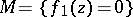, and the dimension ofis, the dimension of the set of critical points is at most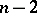.

## Contents

How to Cite This Entry:
Critical point. Encyclopedia of Mathematics. URL: http://encyclopediaofmath.org/index.php?title=Critical_point&oldid=16181
This article was adapted from an original article by E.D. Solomentsev (originator), which appeared in Encyclopedia of Mathematics - ISBN 1402006098. See original article